# Catch up on the latest AI articles# Attack On Multimodal Contrast Learning!

3 main points
✔️ Poisoning backdoor attacks against multimodal contrastive learning models
✔️ Successful poisoning backdoor attack with very low injection rate
✔️ Advocate for the risk of learning from data automatically collected from the Internet

written by Nicholas CarliniAndreas Terzis
(Submitted on 17 Jun 2021)

Subjects: Computer Vision and Pattern Recognition (cs.CV)code：The images used in this article are from the paper, the introductory slides, or were created based on them.

## first of all

Self-supervised learning models, such as Contrastive Learning, can be trained on high-quality unlabeled, noisy data sets. Such learning methods have the advantage that they do not require a high cost of the dataset creation and that learning on noisy data improves the robustness of the learning process.

However, there may be significant risks in training on automatically-collected data without human filtering. In the paper presented in this article, we performed target poisoning and backdoor attacks against multimodal contrastive learning models such as CLIP.

The results show that the attack is successful even with much smaller injection rates compared to standard supervised learning. (This paper is Accept(Oral) in ICLR2022)

### On Multimodal Contrastive Learning

In a typical contrastive learning setting, we learn a function $f:X→E$ that maps inputs to an embedding space such that the embeddings of similar inputs are close and those of different inputs are far apart. While earlier contrast learning-focused mainly on contrast learning in a single domain (e.g., images), multimodal (e.g., images and text) contrast learning methods have started to emerge in recent years.

The paper presented in this article focuses specifically on attacks on image-text multimodal contrast learning models. In this setting, a dataset can be represented as $X \subset A×B (where A is an image and B is a text caption)$.

The goal of the multimodal contrast learning model is then to learn functions $f: A→E,g: B→E$ that map inputs from the respective domains $A, B$ to the German embedding space. For a training sample $(a,b) \in X$, learning is performed to minimize the inner product between the representations $f(a),g(b)$ obtained from each embedding function and maximize the inner product with another sample $(a',b') \in X$. The learned contrast learning model is also usually used for either a feature extractor or a zero-shot classifier.

### Poisoning Backdoor Attacks

In a poisoning attack, a poison train set $X'=X\cupP$ is created by injecting a poison sample $P$ into the train set $X$. Here, targeted poisoning is an attack that injects a poison sample so that some input $X'$ is classified into a specific target label $Y'$.

Also, in a backdoor attack, an image $x'=x \otimes bd$ with a specific trigger (backdoor patch) added to the input is attacked to be classified to a specific target label $y'$.

Here is an example of an image with the backdoor patch added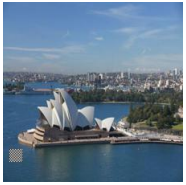(The square-shaped backdoor patch is applied in the lower-left corner of the image.)

In the paper, we perform these targeted poisoning backdoor attacks against multimodal contrastive learning models.

### Attacker Configuration

In the paper, we focus on attacks on the image embedding function $f:X→E$ in multimodal contrastive learning models. We also assume that an attacker can inject a small number of samples into the train set.

However, if the trained contrast training model is used as a feature extractor and as the backbone for additional classifiers, we assume that the train set and algorithms from the training of the classifiers are not accessible.

## Poisoning Backdoor Attacks on Multimodal Contrastive Learning

As the simplest case, we first consider a targeted poisoning attack against a multimodal contrastive learning model. In this case, a targeted poisoning attack can be performed by injecting image-text pairs into the train set that encourage the classification of image $x'$ into target label $y'$.

Specifically, we inject a set of pairs of images $x'$ and a caption sentence associated with the target label $y'$ as a poison sample.

### multisample poisoning attack

If the target image is $x'$ and the target label is $y'$, for the attack on the multimodal contrastive learning model, we need to construct a caption set $Y'$ associated with the label $y'$.

For example, if an image is labeled "basketball", an example caption might be "A photo of a kid playing with a basketball".

If we construct such a caption set $C$, the poison set $P$ injected into the train set is defined as follows.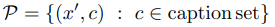In this case, the poison train set is $X'=X\cupP$. The number of poison samples can be controlled by manipulating the size of the caption set (the number of caption sentences). We now consider whether it is practical to inject such a poison set into the train set.

State-of-the-art multimodal contrast learning methods do not manually check the training dataset but filter the training data through automatic cleaning algorithms, such as removing duplicate images. However, this algorithm is not a barrier for attackers, since its purpose is not to defend against attackers but to remove obvious label noise.

If data automatically collected from the Internet is used for training, it is a realistic enough setting for an attacker to inject a poison sample.

### Building a Caption Set

To create a set of caption sentences corresponding to a target label, two main methods are possible. The first method searches for caption sentences containing the target label in the train set and uses the obtained text as it is as a caption sentence. The obtained caption sentences may contain some noise, but it is not a big problem because most of them are correct.

The second way is to use additional information about the model to be attacked, if available.

For example, CLIP uses a specific form of text such as "a photo of a {label}" for label prediction when creating a zero-shot classifier (prompt engineering). Using such prior knowledge, it may be possible to create a caption based on the text format used in prompt engineering, for example.

### Attacks on Contrastive Learning Models

Poisoning attacks on contrastive learning models, unlike attacks on regular supervised models, cannot directly mispredict the model under attack.

The attacker, therefore, takes the form of controlling the embedding function learned by the contrastive learning model and expecting the downstream classification model or zero-shot classifier that uses the embedding to make incorrect predictions. Here, the attacker's goal is to poison the image embedding function $f$ of the control learning model.

On the other hand, since the learning objective of the contrastive learning model is to minimize the inner product of $f_{\theta}(a),g_{\phi}(b)$, an attacker may not necessarily change the behavior of $f$ (only $\phi$ may change). Therefore, by using a diverse set of captions for a single image, we encourage the image embedding function $f$ to be preferentially modified.

### Extension to Backdoor Attacks

When extending targeted poisoning to backdoor attacks, the goal is to cause an image $x$ containing a specific trigger $bd$ to be misclassified.

Therefore, a backdoor attack injects a pair of images $x_i \otimes bd$ containing a particular backdoor pattern $bd$ and a caption associated with the target label. In this case, the poison set is $P=\{(x_i \otimes bd,c) :c \in caption set, x_i \in X_{subset}}$.

## experimental results

We use CLIP for the multimodal contrastive learning model for the attack. The hyperparameters follow the default settings of CLIP. We also use the Conceptual Captions dataset which contains 3 million images.

The results of the targeted poisoning attack are as follows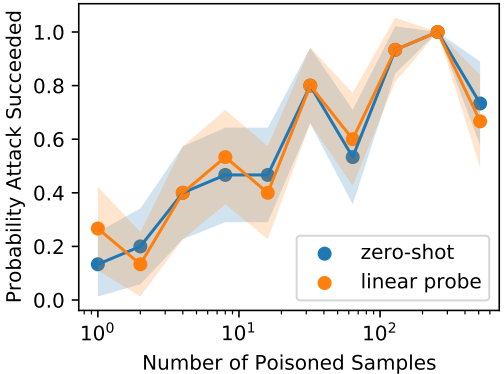In our experiments, we inject a poisoning set consisting of 1-512 samples.

The results show that the attack can be performed with a very low injection rate compared to attacks on general supervised models, achieving a 40% success rate with only three poison samples. We also achieved similar attack success rates in both the zero-shot and linear probe settings. The results of the backdoor attack are also shown below.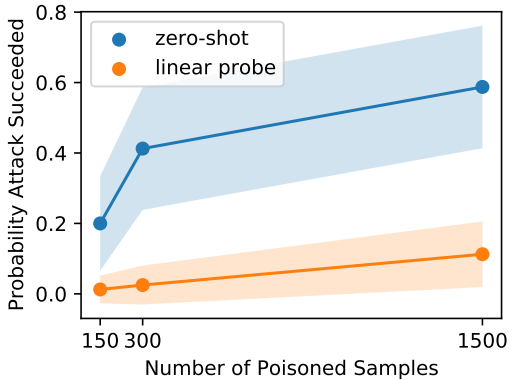Experiments have been conducted at three injection rates of 0.0005%, 0.01%, and 0.05%. Unlike the poisoning case, the attack success rate is smaller in the linear probe setting, but for zero-shot, we achieved an attack success rate of about 50% even with an injection rate as low as 0.01%. To measure the effectiveness of the backdoor attack, we also introduce a metric called "backdoor Z-Score", which is defined as the relative similarity between the embeddings of two images with backdoor patches applied.

If the backdoor attack is working effectively, the value of this metric will be higher. Here are the results for varying the model size and dataset size.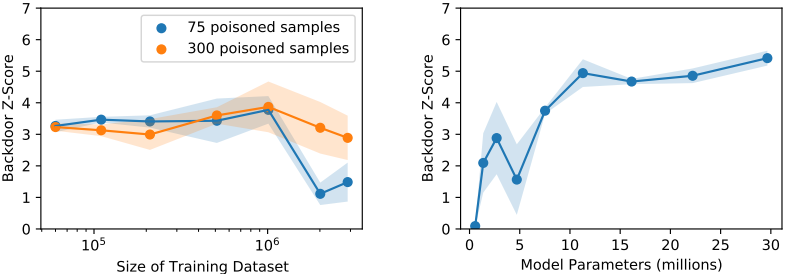When the size of the dataset is varied under the fixed number of poison samples, we find that the attack success rate remains almost unchanged. However, when the dataset size exceeds 1 million, the attack success rate changes. It is also shown that the attack success rate tends to increase as the number of parameters in the model increases.

## summary

In this article, we presented our work on a poisoning backdoor attack against a multimodal continuous learning model. Experimental results showed that the backdoor and poisoning attacks achieved a success rate of over 40% even with an injection rate of 0.01% and 0.0001%, respectively, indicating the potential risk of using images automatically collected from the Internet for training.

If you have any suggestions for improvement of the content of the article,